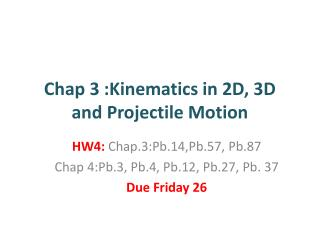Download PresentationChap 3 :Kinematics in 2D, 3D and Projectile Motion

Chap 3 :Kinematics in 2D, 3D and Projectile Motion - PowerPoint PPT Presentation

Chap 3 :Kinematics in 2D, 3D and Projectile Motion. HW4 : Chap.3:Pb.14,Pb.57, Pb.87 Chap 4:Pb.3, Pb.4, Pb.12, Pb.27, Pb . 37 Due Friday 26. Unit Vectors. Unit vectors have magnitude 1 . Using unit vectors, any vector can be written in terms of its components:. Vector Kinematics.I am the owner, or an agent authorized to act on behalf of the owner, of the copyrighted work described.
Download PresentationChap 3 :Kinematics in 2D, 3D and Projectile Motion

Download Policy: Content on the Website is provided to you AS IS for your information and personal use and may not be sold / licensed / shared on other websites without getting consent from its author.While downloading, if for some reason you are not able to download a presentation, the publisher may have deleted the file from their server.

- - - - - - - - - - - - - - - - - - - - - - - - - - E N D - - - - - - - - - - - - - - - - - - - - - - - - - -
Presentation Transcript
1. Chap 3 :Kinematics in 2D, 3D and Projectile Motion HW4: Chap.3:Pb.14,Pb.57, Pb.87 Chap 4:Pb.3, Pb.4, Pb.12, Pb.27, Pb. 37 Due Friday 26

2. Unit Vectors Unit vectors have magnitude 1. Using unit vectors, any vector can be written in terms of its components:

3. Vector Kinematics In two or three dimensions, the displacement is a vector:

4. Vector Kinematics As Δt and Δr become smaller and smaller, the average velocity approaches the instantaneous velocity.

5. Vector Kinematics The instantaneous acceleration is in the direction of Δ= 2 –1, and is given by:

6. Vector Kinematics Using unit vectors,

7. Example 3-5: Position given as a function of time

8. Vector Kinematics Generalizing the one-dimensional equations for constant acceleration:

9. Projectile Motion Aprojectileis an object moving in two dimensions under the influence of Earth's gravity; its path is a parabola.

10. 2D motion = two independent 1D motions Figure from “Conceptual Physics for Everyone”, Paul G. Hewitt, Addison Wesley, 2002.

11. Projectile Motion It can be understood by analyzing the horizontalandverticalmotions separately.

12. Projectile Motion Thespeedin the x-direction is constant; in the y-direction the object moves with constant accelerationg. This photograph shows two balls that start to fall at the same time. The one on the right has an initial speed in the x-direction. It can be seen that vertical positions of the two balls are identical at identical times, while the horizontal position of the yellow ball increases linearly.

13. X and Y are Independent • Red ball is dropped vix=viy=0 • White ball is tossed horizontally viy=0 vix≠0 • Yellow lines show equal time intervals.

14. Projectile Motion If an object is launchedat an initial angle of θ0 with the horizontal, the analysis is similar except that the initial velocity has a verticalcomponent.

15. vi viy viy vix Projectile Motion ay=g ax=0 y  x

16. Solving Problems Involving Projectile Motion Projectilemotion is motion with constant acceleration in two dimensions, where the acceleration is g and is down.

17. Solving Problems Involving Projectile Motion • Readthe problem carefully, and choose the object(s) you are going to analyze. • Draw a diagram. • Choosean origin and a coordinate system. • Decideon the time interval; this is the same in both directions, and includes only the time the object is moving with constant acceleration g. • Examinethe x and y motions separately.

18. Solving Problems Involving Projectile Motion 6. List known and unknown quantities. Remember that vx never changes, and that vy = 0 at the highest point. 7. Planhow you will proceed. Use the appropriate equations; you may have to combine some of them.

19. Solving Problems Involving Projectile Motion Example 3-6: Driving off a cliff. A movie stunt driver on a motorcycle speeds horizontally off a 50.0-m-high cliff. How fast must the motorcycle leave the cliff top to land on level ground below, 90.0 m from the base of the cliff where the cameras are? Ignore air resistance.

20. Solving Problems Involving Projectile Motion Example 3-7: A kicked football. A football is kicked at an angle θ0 = 37.0° with a velocity of 20.0 m/s, as shown. Calculate (a) the maximum height, (b) the time of travel before the football hits the ground, (c) how far away it hits the ground, (d) the velocity vector at the maximum height, and (e) the acceleration vector at maximum height. Assume the ball leaves the foot at ground level, and ignore air resistance and rotation of the ball.